• 2019-11-10

• 2019-10-08

• 2019-10-03

• 2019-10-03

• 2019-10-02

## 教师风采｜为师者点赞！

“^_^”、“*_*”、“^o^”、“^_~”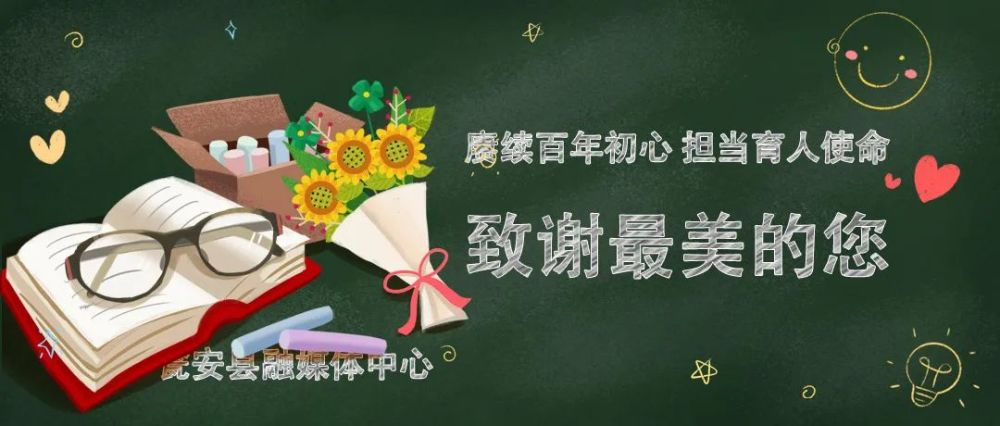“^_^”、“*_*”、“^o^”、“^_~”

“^_^”、“*_*”、“^o^”、“^_~”

“^_^”、“*_*”、“^o^”、“^_~”

“^_^”、“*_*”、“^o^”、“^_~”

“^_^”、“*_*”、“^o^”、“^_~”

“^_^”、“*_*”、“^o^”、“^_~”

“^_^”、“*_*”、“^o^”、“^_~”

“^_^”、“*_*”、“^o^”、“^_~”

“^_^”、“*_*”、“^o^”、“^_~”

“^_^”、“*_*”、“^o^”、“^_~”

“^_^”、“*_*”、“^o^”、“^_~”

“^_^”、“*_*”、“^o^”、“^_~”“^_^”、“*_*”、“^o^”、“^_~”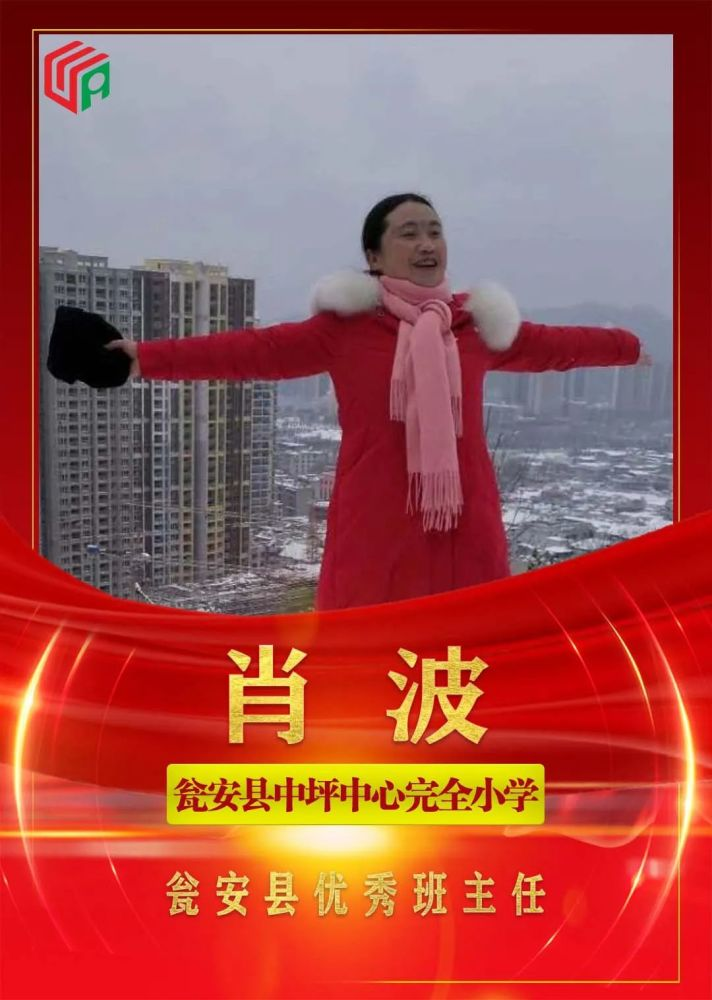“^_^”、“*_*”、“^o^”、“^_~”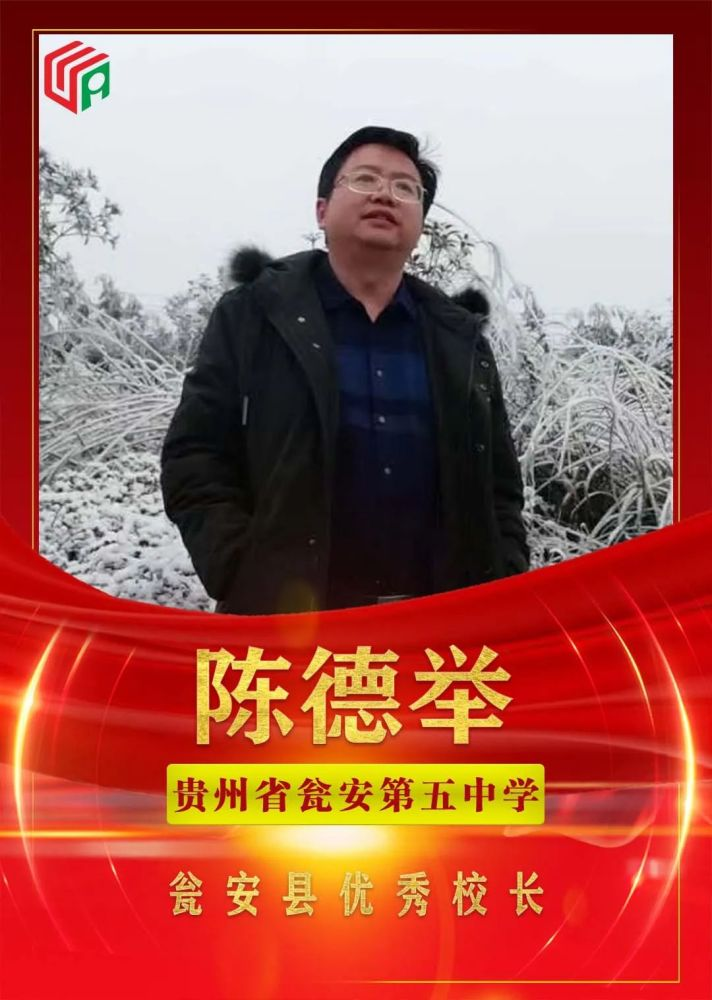“^_^”、“*_*”、“^o^”、“^_~”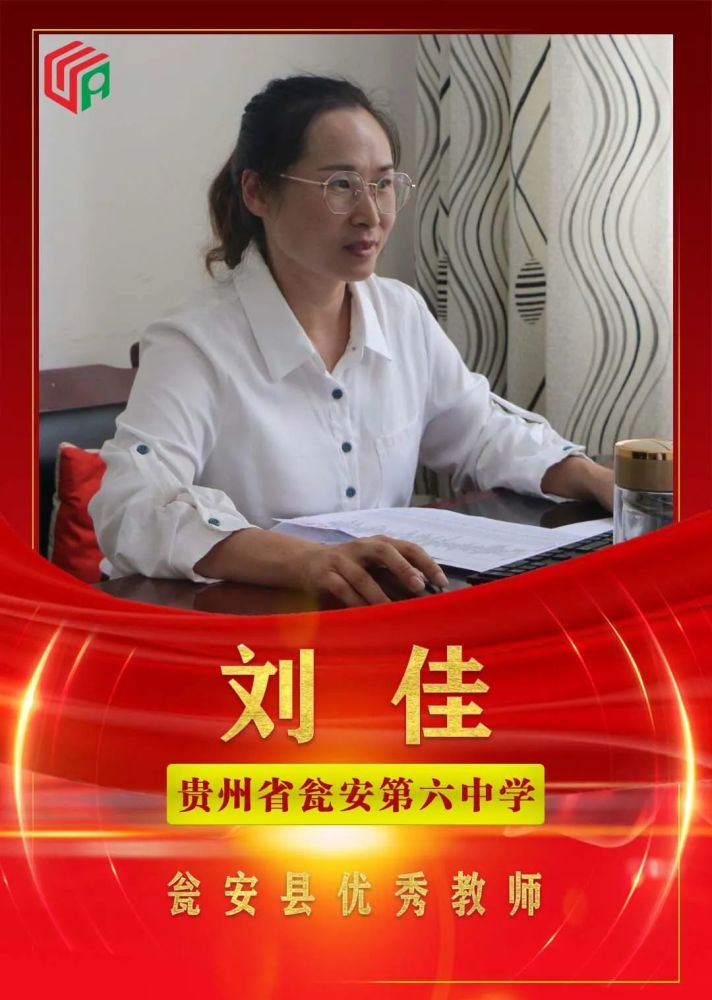“^_^”、“*_*”、“^o^”、“^_~”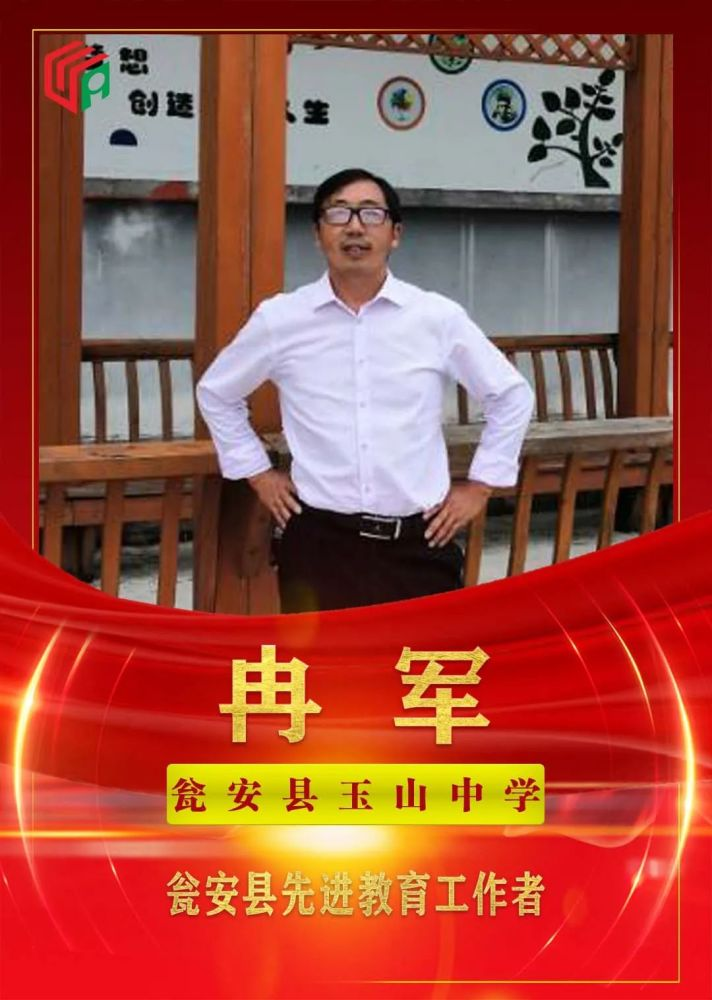“^_^”、“*_*”、“^o^”、“^_~”

“^_^”、“*_*”、“^o^”、“^_~”

“^_^”、“*_*”、“^o^”、“^_~”

“^_^”、“*_*”、“^o^”、“^_~”

“^_^”、“*_*”、“^o^”、“^_~”

“^_^”、“*_*”、“^o^”、“^_~”## 瓮安县

“^_^”、“*_*”、“^o^”、“^_~”自己的学生,九月的瓮安大地他们的身影，美得不可方物他们忠诚于教育事业志存高远，勤恳敬业闪耀着矢志不渝的信仰之美他们执着于授业解惑循循善诱，因材施教闪耀着一往无前的奋斗之美他们倾情于春风化雨关心呵护，童心不泯闪耀……

2021-09-15 19:49

2021-09-15 19:49

2021-09-15 19:48

2021-09-15 19:44

2021-09-15 19:43

2021-09-15 19:42